# Graphics CSCI 343 Fall 2019 Lecture 28 Scan

• Slides: 12
Download presentation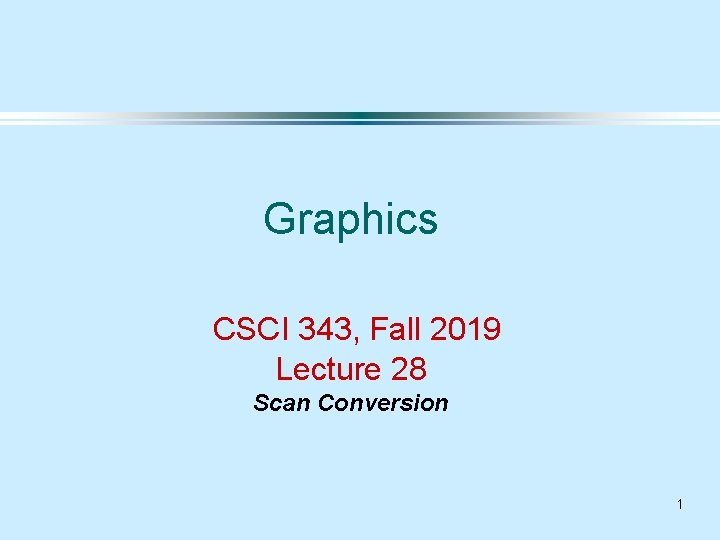Graphics CSCI 343, Fall 2019 Lecture 28 Scan Conversion 1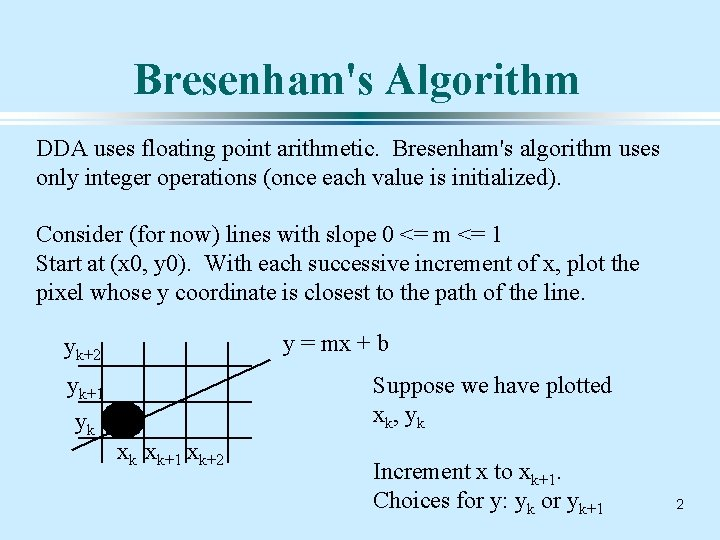Bresenham's Algorithm DDA uses floating point arithmetic. Bresenham's algorithm uses only integer operations (once each value is initialized). Consider (for now) lines with slope 0 <= m <= 1 Start at (x 0, y 0). With each successive increment of x, plot the pixel whose y coordinate is closest to the path of the line. y = mx + b yk+2 yk+1 yk Suppose we have plotted xk, yk xk xk+1 xk+2 Increment x to xk+1. Choices for y: yk or yk+1 2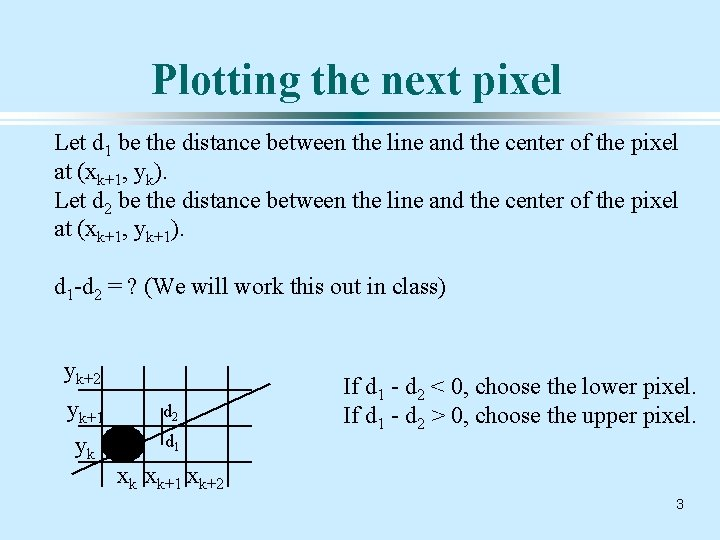Plotting the next pixel Let d 1 be the distance between the line and the center of the pixel at (xk+1, yk). Let d 2 be the distance between the line and the center of the pixel at (xk+1, yk+1). d 1 -d 2 = ? (We will work this out in class) yk+2 yk+1 yk d 2 d 1 If d 1 - d 2 < 0, choose the lower pixel. If d 1 - d 2 > 0, choose the upper pixel. xk xk+1 xk+2 3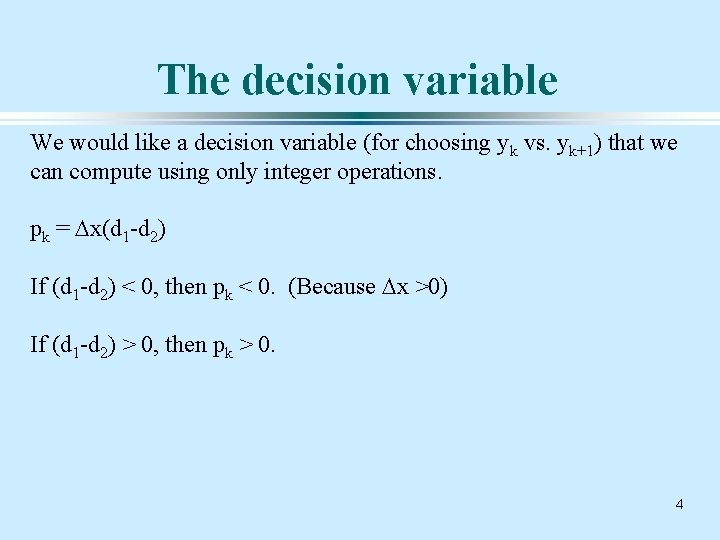The decision variable We would like a decision variable (for choosing yk vs. yk+1) that we can compute using only integer operations. pk = Dx(d 1 -d 2) If (d 1 -d 2) < 0, then pk < 0. (Because Dx >0) If (d 1 -d 2) > 0, then pk > 0. 4Incrementally computing pk If we know pk, we would like to calculate pk+1 using only integer computations. pk = Dx(d 1 -d 2) What is pk in terms of Dx, Dy, xk, yk? We will work this out in class. What is the difference between pk+1 and pk? 5Calculating the Starting pk If we know pk, we can compute pk+1 using only integer computations. To start, we must calculate p 0. (We will work this out in class). pk = 2 Dyxk - 2 Dxyk + (2 Dy + Dx(2 b -1)) 6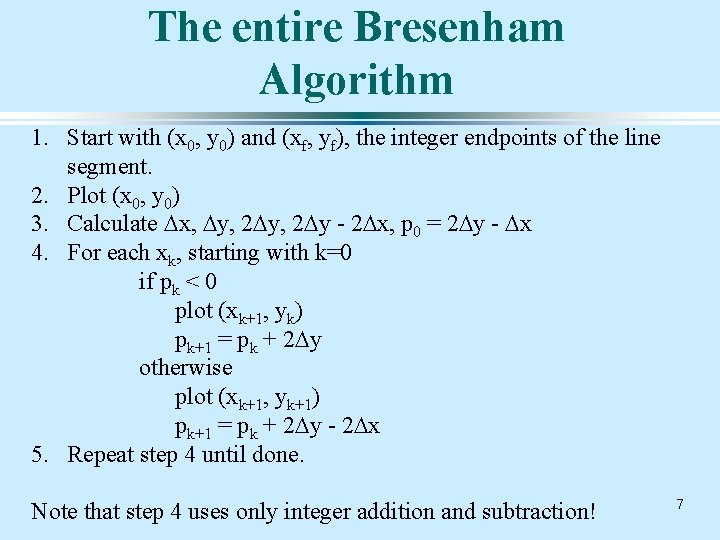The entire Bresenham Algorithm 1. Start with (x 0, y 0) and (xf, yf), the integer endpoints of the line segment. 2. Plot (x 0, y 0) 3. Calculate Dx, Dy, 2 Dy - 2 Dx, p 0 = 2 Dy - Dx 4. For each xk, starting with k=0 if pk < 0 plot (xk+1, yk) pk+1 = pk + 2 Dy otherwise plot (xk+1, yk+1) pk+1 = pk + 2 Dy - 2 Dx 5. Repeat step 4 until done. Note that step 4 uses only integer addition and subtraction! 7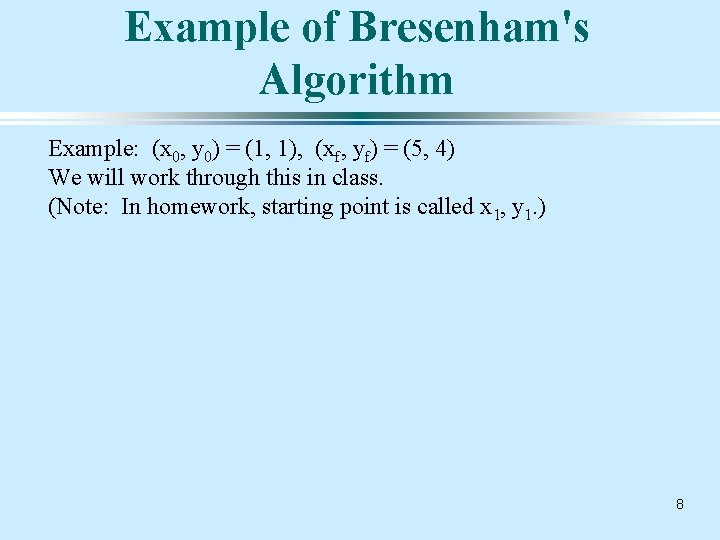Example of Bresenham's Algorithm Example: (x 0, y 0) = (1, 1), (xf, yf) = (5, 4) We will work through this in class. (Note: In homework, starting point is called x 1, y 1. ) 8Scan Conversion of Polygons Inside-Outside Testing: How do you know whether to shade a pixel as inside or outside the polygon? (xmax, ymax) Simple case: (xmin, ymin) if (x >= xmin) && (x<=xmax) && (y>= ymin) && (y <= ymax) { draw. Pixel(x, y, inside_color); } else { draw. Pixel(x, y, outside_color); } 9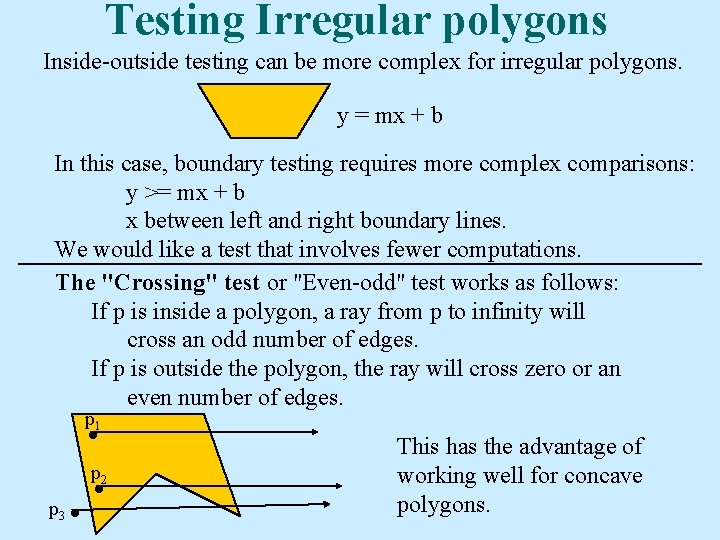Testing Irregular polygons Inside-outside testing can be more complex for irregular polygons. y = mx + b In this case, boundary testing requires more complex comparisons: y >= mx + b x between left and right boundary lines. We would like a test that involves fewer computations. The "Crossing" test or "Even-odd" test works as follows: If p is inside a polygon, a ray from p to infinity will cross an odd number of edges. If p is outside the polygon, the ray will cross zero or an even number of edges. p 1 p 2 p 3 This has the advantage of working well for concave polygons.Winding number Suppose the polygon is complex. How do we compute inside and outside? Crossing test Winding Number Compute the winding number. Traverse the polygon in one direction. The number of times you go around point p is the winding number. Define inside as the region for which the winding number is not 11 zero.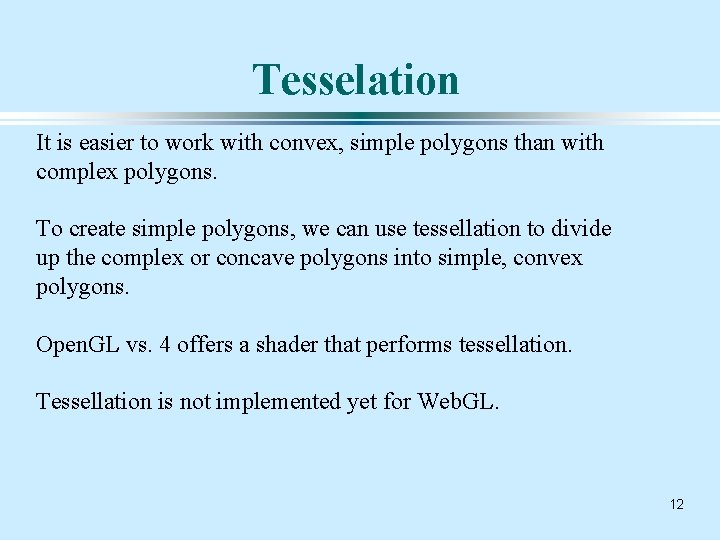Tesselation It is easier to work with convex, simple polygons than with complex polygons. To create simple polygons, we can use tessellation to divide up the complex or concave polygons into simple, convex polygons. Open. GL vs. 4 offers a shader that performs tessellation. Tessellation is not implemented yet for Web. GL. 12